## Regular Polyhedron

A polyhedron is said to be regular if its Faces and Vertex Figures are Regular (not necessarily Convex) polygons (Coxeter 1973, p. 16). Using this definition, there are a total of nine regular polyhedra, five being the Convex Platonic Solids and four being the Concave (stellated) Kepler-Poinsot Solids. However, the term regular polyhedra'' is sometimes used to refer exclusively to the Convex Platonic Solids.

It can be proven that only nine regular solids (in the Coxeter sense) exist by noting that a possible regular polyhedron must satisfyGordon showed that the only solutions to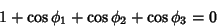of the form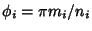are the permutations ofand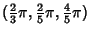. This gives three permutations of (3, 3, 4) and six of (3, 5,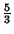) as possible solutions to the first equation. Plugging back in gives the Schläfli Symbols of possible regular polyhedra as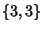,,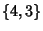,,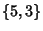,,,, and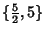(Coxeter 1973, pp. 107-109). The first five of these are the Platonic Solids and the remaining four the Kepler-Poinsot Solids.

Every regular polyhedron hasaxes of symmetry, whereis the number of Edges, andPlanes of symmetry, whereis the number of sides of the corresponding Petrie Polygon.

See also Convex Polyhedron, Kepler-Poinsot Solid, Petrie Polygon, Platonic Solid, Polyhedron, Polyhedron Compound, Sponge, Vertex Figure

References

Coxeter, H. S. M. Regular and Semi-Regular Polytopes I.'' Math. Z. 46, 380-407, 1940.

Coxeter, H. S. M. Regular Polytopes, 3rd ed. New York: Dover, pp. 1-17, 93, and 107-112, 1973.

Cromwell, P. R. Polyhedra. New York: Cambridge University Press, pp. 85-86, 1997.